GFG App
Open AppBrowser
Continue

# Class 12 RD Sharma Solutions – Chapter 30 Linear Programming – Exercise 30.4 | Set 1

### Question 1. If a young man drives his vehicle at 25 km/hr, he has to spend Rs 2 per km on petrol. If he drives it at a faster speed of 40 km/hr, the petrol cost increases to Rs 5/per km. He has Rs 100 to spend on petrol and travel within one hour. Express this as an LPP and solve the same.

Solution:

Assume the young man drives x km at a speed of 25 km/hr and y km at a speed of 40 km/hr.

Thus, x, y ≥ 0

Given: If he spends Rs 2/km if he drives at a speed of 25 km/hr

Rs 5/km if he drives at a speed of 40 km/hr.

Hence,

Money spent by him when he traveled x km and y km will be Rs 2x and Rs 5y respectively.

Thus, here it is given that he can spend Rs100 maximum.

Therefore,

2x + 5y ≤ 100

So, for traveling with a speed of 25 km/hr the time that he will be spending = x/25 hr

So, for traveling with a speed of 40km/hr the time that he will be spending = y/40 hr

And also,

The time he is having is 1 hour.

x/25 + y/40 ≤ 1

40x + 25y ≤ 1000

The distance covered Z = x + y

That is to be maximized.

Therefore, the formula of the given linear programming problem is

Max Z = x + y

Subject to constraints

2x + 5y ≤ 100

40x + 25y ≤ 1000

x, y ≥ 0

First we will convert in-equations as follows:

2x + 5y = 100

40x + 25y = 1000

x = 0 and y = 0.

The area shown by 2x + 5y ≤ 100

The line 2x + 5y = 100 connects the coordinate axes at A(50, 0) and B(0, 20) respectively.

After connecting these points, we will get the line 2x + 5y = 100.

Thus, (0, 0) assure the 2x + 5y = 100.

As, the area that consists the origin shows the solution set of the in-equation 2x + 5y ≤ 100

The area shown by 40x + 25y ≤ 1000

The line 40x + 25y = 1000 connects the coordinate axes at C(25, 0) and D(0, 40) respectively.

After connecting these points, we will get the line 2x + y = 12.

Thus, (0, 0) assure the 40x + 25y = 1000.

As, the area that consists of the origin shows the solution set of the in-equation 40x + 25y ≤ 1000

The area shown by x ≥ 0,

y ≥ 0 :

Since, all the points in the first quadrant assure these in-equations.

As, the first quadrant is the area shown by the in-equations x ≥ 0

and

y ≥ 0.

The suitable area is determined by the system of constraints

2x + 5y ≤ 100,

40x + 25y ≤ 1000,

x ≥ 0

and

y ≥ 0 are as follows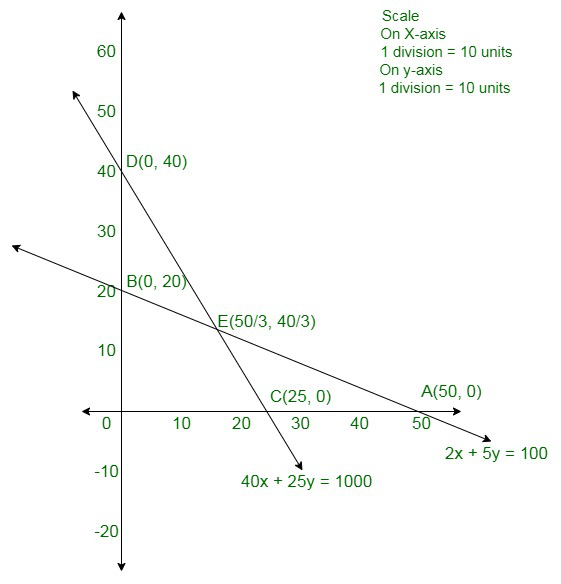The corner points are O(0, 0), B(0, 20), E(50/3, 40/3), and C(25, 0). The value of Z at these corner points are as follows:

The maximum value of Z is 30 that is acquired at E.

Therefore,

The maximum distance traveled by the young man is 30 kms, if he drives 50/3 km at a speed of 25 km/hr and 40/3 km at a speed of 40 km/hr.

### He makes a profit of Rs 6.00 on item A and Rs 4.00 on item B. Assuming that he can sell all that he produces, how many of each item should he produces so as to maximize his profit? Determine his maximum profit. Formulate this LPP mathematically and then solve it.

Solution:

Assume x units of item A and y units of item B be produced.

Therefore, x, y ≥ 0.

Given:

Machines I and II are efficient of being operated for at most 12 hours whereas Machine III should operate at least for 5 hours a day.

As per in the question,

The constraints are

x + 2y ≤ 12

2x + y ≤ 12

x + 5/4

y ≥ 5

He makes a profit of Rs 6.00 on item A and Rs. 4.00 on item B. Profit made by him in manufacturing x items of A and y items of B is 6x + 4y.

Total profit is Z

Z = 6x + 4y that is to be maximized

Therefore, the formula of the given linear programming problem is

Max Z = 6x + 4y,

Subject to constraints

x + 2y ≤ 12

2x + y ≤ 12

x + 5/4

y ≥ 5x,

y ≥ 0

First, we have to convert the in-equations into equations as follows:

x + 2y = 12,

2x + y = 12,

x + 5/4

y = 5,

x = 0 and

y = 0.

The area shown by x + 2y ≤ 12

The line x + 2y = 12 connects the coordinate axes at A(12, 0) and B(0, 6) respectively.

After connecting these points, we will get the line x + y = 12.

As (0, 0) assure the x + 2y = 12.

As, the area that consists the origin shows the solution set of the in-equation x + 2y ≤ 12

The area shown by 2x + y ≤ 12

The line 2x + y = 12 connects the coordinate axes at C(6, 0) and D(0, 12) respectively.

After connecting these points, we will connect the line 2x + y = 12.

As (0, 0) assure the 2x + y = 12.

As, the area that consists the origin shown the solution set of the in-equation 2x + y ≤ 12

The area shown by x + 5/4y ≥ 5

The line x + 5/4y ≥ 5 connects the coordinate axes at E(5, 0) and F(0, 4) respectively.

After connecting these points,

We will get the line x + 5/4y = 5.

As (0, 0) assure the x + 5/4y ≥ 5.

As, the area that does not consist the origin shows the solution set of the in-equation x + 5/4y ≥ 5

The area shown by x ≥ 0,

y ≥ 0 :

As all the points in the first quadrant assure these in-equations.

Thus, the first quadrant is the area shown by the in-equations x ≥ 0

and

y ≥ 0.

The suitable area determined by the system of constraints

x + 2y ≤ 12,

2x + y ≤ 12,

x + 5/4y ≥ 5, x,

y ≥ 0 are as follows.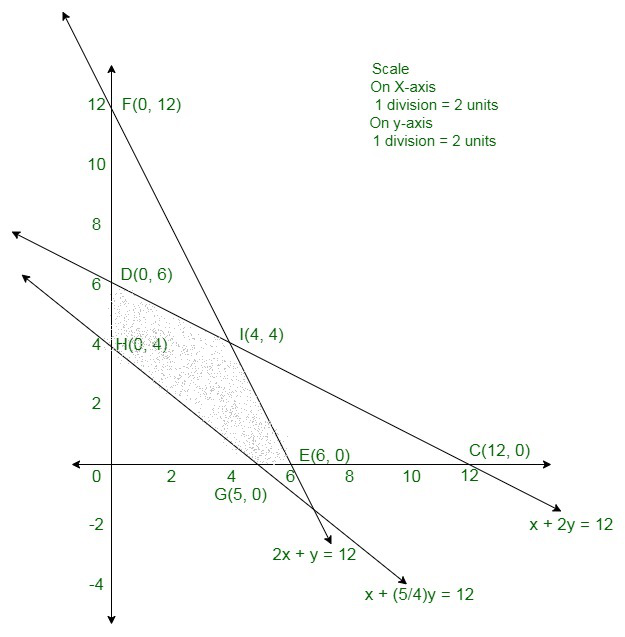Therefore, the maximum profit is of Rs 40 gained when 4 units each of item A and B are manufactured.

The corner points are D(0, 6), I(4, 4), C(6, 0), G(5, 0), and H(0, 4).

The values of Z at these corner points are as follows:

Hence, the maximum value of Z is 40 which is acquired at I(4, 4).

### Question 3. Two tailors, A and B earn Rs 15 and Rs 20 per day respectively. A can stitch 6 shirts and 4 pants while B can stitch 10 shirts and 4 pants per day. How many days shall each work if it is desired to produce atleast 60 shirts and 32 pants at a minimum labour cost?

Solution:

Assume that tailor A work for x days and tailor B work for y days.

In a day; A can stitch 6 shirts and 4 pants whereas B can stitch 10 shirts and 4 pants.

Therefore, in x days; A can stitch 6x shirts and 4x pants whereas in y days B can stitch 10y shirts and 4y pants.

Given: The minimum need of the shirt and pants are respectively 60 and 32.

Therefore,

6x + 10y ≥ 60

4x + 4y ≥ 32

So further, it is given that A and B earn Rs 15 and Rs 20 per day respectively.

Therefore, A earn Rs 15x and B earns Rs 20y.

Assume the total cost is Z

Z = 15x + 20y

As days cannot be negative.

x, y ≥ 0 (always)

Min Z = 15x + 20y

Subject to constraints

6x + 10y ≥ 60

4x + 4y ≥ 32

x, y ≥ 0

First we will convert in-equations into equations as follows:

6x + 10y = 60,

4x + 4y = 32,

x = 0,

y = 0

The area shown by 6x + 10y ≥ 60

The line 6x + 10y = 60 connects the coordinate axes at A(10,0) and B(0,6) respectively.

After connecting these points we will get the line 6x + 10y = 60.

As, (0, 0) assure the 6x + 10y ≥ 60.

Since, the area that does not consist the origin shown the solution set of the in-equation 6x + 10y ≥ 60

Area shown by 4x + 4y ≥ 32

The line 4x + 4y = 32 connects the coordinate axes at C(8,0) and D(0,8) respectively.

After connecting these points we will get the line 4x + 4y = 32.

As, (0, 0) assure the 4x + 4y ≥ 32.

As, the area that does not consist the origin shows the solution set of the in-equation 4x + 4y ≥ 32.

The area shown by x ≥ 0,

y ≥ 0 :

Since, all the points in the first quadrant assure these in-equations.

As, the first quadrant is the area shown by the in-equations x ≥ 0

and

y ≥ 0.

The suitable area determined by the system of constraints 6x + 10y ≥ 60,

4x + 4y ≥ 32

x, y ≥ 0 are as follows.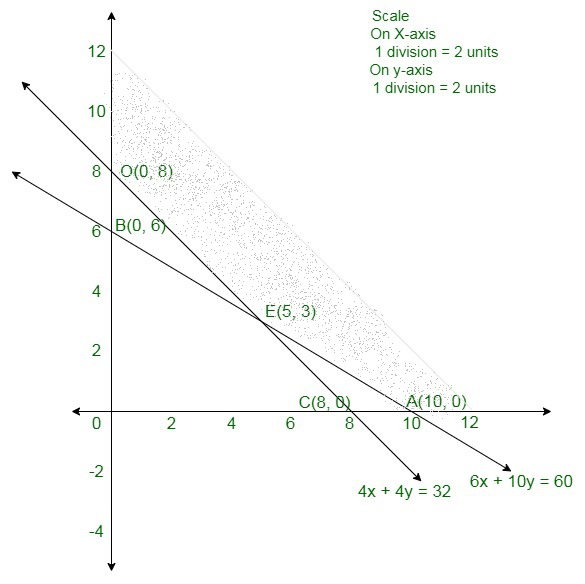The corner points are D(0, 8), E(5, 3), A(10, 0).

The values of Z at these corner points are as follows:

The minimum value of Z is 135 which is acquired at E(5, 3).

Therefore, For minimum labor cost, A must work for 5 days and B must work for 3 days.

### Question 4. A factory manufactures two types of screws, A and B, each type requiring the use of two machines – an automatic and a hand-operated. It takes 4 minute on the automatic and 6 minutes on the hand-operated machines to manufacture a package of screws ‘A’, while it takes 6 minutes on the automatic and 3 minutes on the hand-operated machine to manufacture a package of screws ‘B’. Each machine is available for at most 4 hours on any day. The manufacturer can sell a package of screws ‘A’ at a profit of 70 P and screws ‘B’ at a profit of Rs 1. Assuming that he can sell all the screws he can manufacture, how many packages of each type should the factory owner produce in a day in order to maximize his profit? Determine the maximum profit.

Solution:

Assume the factory manufacture x screws of type A and y screws of type B on each day,

Thus,

x, y ≥  0.

Given information in a table as;

4x + 6y ≤ 240

6x + 3y ≤ 240

The manufacturer can sell a package of screws ‘A’ at a profit of Rs 0.7 and screws ‘B’ at a profit of Re 1.

Assume the total profit is Z,

Z = 0.7x + 1y

Therefore, the formula of the given linear programming problem is

Maximize Z = 0.7x + 1y

Subject to the constraints,

4x + 6y ≤ 240

6x + 3y ≤ 240

x, y ≥ 0

First, we will convert the in-equations into equations as follows:

4x + 6y = 240, 6x + 3y = 240, x = 0, y = 0.

Area shown by 4x + 6y ≥  240

The line 4x + 6y = 240 connects the coordinate axes at A(60, 0) and B(0, 40) respectively.

After connecting these points we will get the line 4x + 6y = 240.

As, (0, 0) assure the 4x + 6y ≥  240.

As, the area that consists the origin shows the solution set of the in-equation 4x + 6y ≥  240.

The area shown by 6x + 3y ≥  240

The line 6x + 3y = 240 connects the coordinate axes at C(40, 0) and d(0, 80) respectively.

After connecting these points we will get the line 6x + 3y = 240.

As, (0, 0) assure the 6x + 3y ≥  240.

Since, the area that consist the origin shows the solution set of the in-equation 6x + 3y ≥  240.

The area shown by

x ≥  0,

y ≥ 0 :

As, all the points in the first quadrant assure these in-equations.

Since, the first quadrant is the area shown by the in-equations

x ≥  0

and

y ≥  0.

The suitable are determined by the system of constraints

4x + 6y ≤ 240,

6x + 3y ≤ 240,

x ≥  0,

y ≥  0 are as follows.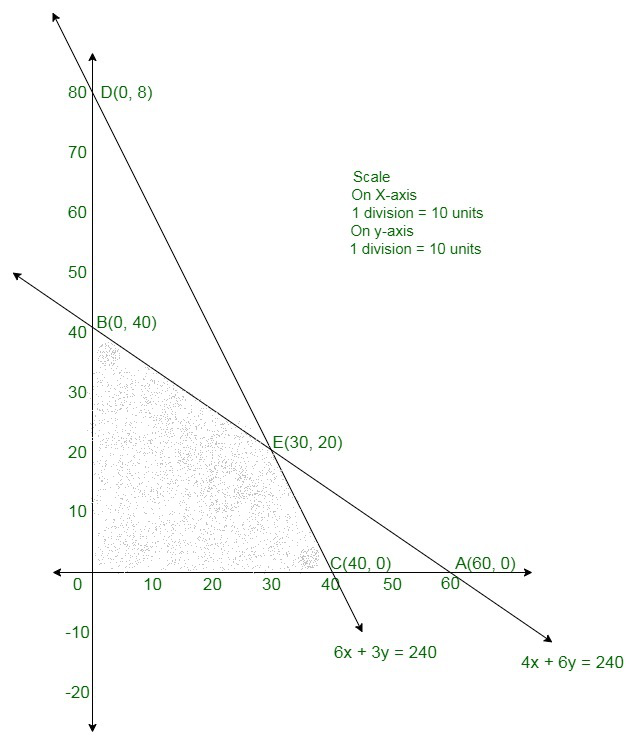The corner points are C(40, 0), E(30, 20), B(0, 40). The values of Z at these corner points are as follows

The maximum value of Z is 410 at (30, 20).

Therefore, the factory must manufacture 30 packages of screws A and 20 packages

of screws b to get the maximum profit of Rs 410.

### Question 5. A company produces two types of leather belts, say type A and B. Belt A is a superior quality and belt B is of a lower quality. Profits on each type of belt are Rs 2 and Rs 1.50 per belt, respectively. Each belt of type A requires twice as much time as required by a belt of type B. If all belts were of type B, the company could produce 1000 belts per day. But the supply of leather is sufficient only for 800 belts per day (both A and B combined). Belt A requires a fancy buckle and only 400 fancy buckles are available for this per day. For belt of type B, only 700 buckles are available per day. How should the company manufacture the two types of belts in order to have a maximum overall profit?

Solution:

Assume the company manufactures x belts of types A and y belts of type B.

Number of belts cannot be negative.

Thus,

x, y ≥ 0 (always)

It is given that leather is enough only for 800 belts per day (both A and B combined).

Thus,

x + y ≤ 800

It is given that the rate of manufacture of belts of type B is 1000 per day.

Therefore, the time taken to produce y belts of type B is y/1000.

And,

As each belt of type A needs twice as much time as a belt of type B, the rate of production of belts of type A is 500 per day and thus, total time taken to produce x belts of type A is x/500

Therefore,

We have,

x/500 + y/1000 ≤ 1

Or,

2x + y ≤ 1000

Belt A needs fancy buckle and only 400 fancy buckles are accessible for this per day.

x ≤ 400

For Belt of type B only 700 buckles are accessible per day.

y ≤ 700

Profits on each type of belt are Rs 2 and Rs 1.50 per belt, respectively.

Thus, profit gained on x belts of type A and y belts of type B is Rs 2x and Rs 1.50y respectively.

Therefore, the total profit would be Rs(2x + 1.50y).

Assume Z denote the total profit

Z = 2x + 1.50y

Therefore, the mathematical formulation of the given linear programming problem is;

Max Z = 2x + 1.50y subject to

x + y ≤ 800

2x + y ≤ 1000

x ≤ 400

y ≤ 700

First we will convert these in-equations into equations as follows:

x + y = 800

2x + y = 1000

x = 400

y = 700

The area shown by x + y = 800

The line x + y = 800 meets the coordinate axes at A(800, 0) and B(0, 800) respectively.

After connecting these points we obtain the line x + y = 800.

As (0, 0) assure the x + y ≥ 800.

As, the area that consist the origin shows the solution set of the in-equation x + y ≥ 800.

The area shown by 2x + y ≥  1000

The line 2x + y = 1000 connects the coordinate axes at C(500, 0) and D(0, 1000) respectively.

After connecting these points we will get the line 2x + y = 1000.

As, (0, 0) assure the 2x + y ≥ 1000.

As, the area that consists the origin shows the solution set of the in-equation 2x + y ≥ 1000.

The area shown by x ≤ 400

The line x = 400 will pass through (400, 0).

The area to the left of the line x = 400 will assure the in-equation x ≤ 400

The area shown by y ≤ 700

The line y = 700 will pass through (0, 700).

The area to the left of the line y = 700 will assure the in-equation y ≤ 700.

The area shown by x ≥ 0,

y ≥ 0 :

As, all the points in the first quadrant assure these in-equations.

As, the first quadrant is the area shown by the in-equations x ≥ 0

and y ≥ 0.

The suitable area determined by the system of constraints x + y ≤ 800,

2x + y ≤ 1000,

x ≤ 400,

y ≤ 700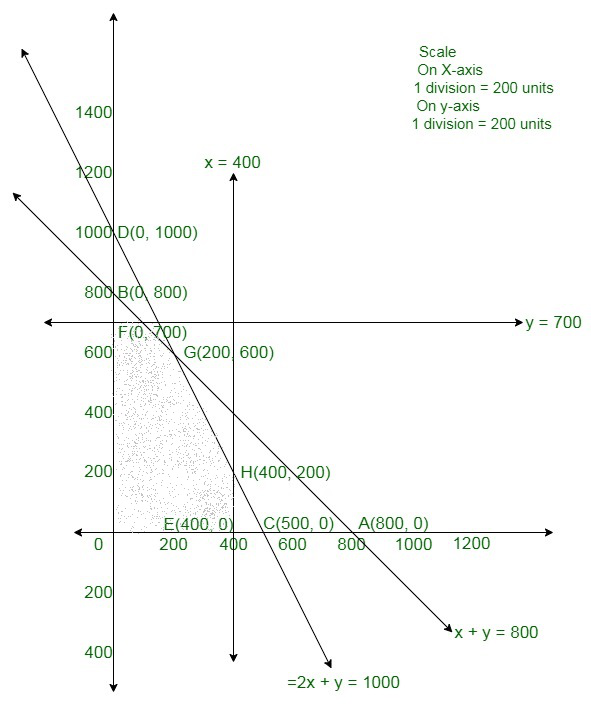The corner points are F(0, 700), G(200, 600), H(400, 200), E(400, 0).

The values of Z at these corner points are as follows

The maximum value of Z is 1300 which is attained at G(200, 600).

Therefore, the maximum profit acquires is Rs 1300 when 200 belts of type A and 600 belts of type B are produced.

### Question 6. A small manufacturer has employed 5 skilled men and 10 semi-skilled men and makes an article in two qualities deluxe model and anordinary model. The making of a deluxe model requires 2 hrs. work by a skilled man and 2 hrs. work by a semi-skilled man. The ordinarymodel requires 1 hr by a skilled man and 3 hrs. by a semi-skilled man. By union rules no man may work more than 8 hrs per day. The manufacturers clear profit on deluxe model is Rs 15 and on an ordinary model is Rs 10. How many of each type should be made in order to maximize his total daily profit.

Solution:

Assume x articles of deluxe model and y articles of an ordinary model be made.

Numbers cannot be negative.

Therefore,

x, y ≥ 0 (always)

As per the question,

The profit on each model of deluxe and ordinary type model are Rs 15 and Rs 10.

Thus, profits on x deluxe model and y ordinary models are 15x and 10y.

Assume Z be total profit,

So,

Z = 15x + 10y

As, the making of a deluxe and ordinary model needs 2 hrs. and 1 hr work by skilled men,

so, x deluxe and y ordinary models needs 2x and y hours of skilled men but time needed

by skilled men is 5 × 8 = 40 hours.

Thus,

2x + y ≤ 40 {First Constraint}

As, the making of a deluxe and ordinary model needs 2 hrs. and 3 hrs work by semi-skilled men,

Since, x deluxe and y ordinary models need 2x and 3y hours of skilled men but time

need by skilled men is 10 × 8 = 80 hours.

As,

2x + 3y ≤ 80 {Second constraint}

Therefore, the formula of the given linear programming problem is

Max Z = 15x + 10y

Subject to constraints,

2x + y ≤ 40

2x + 3y ≤ 80

x, y ≥ 0

Area 2x + y ≤ 40:

Line 2x + 4y = 40 connects axes at

A1(20, 0), B1(0, 40) respectively.

The area having origin shows 2x + 3y ≤ 40 as (0, 0) assure 2x + y ≤ 40

Area 2x + 3y ≤ 80:

Line 2x + 3y = 80 connects axes at

A2(40, 0), B2(0, 80/3) respectively.

The area having origin shows 2x + 3y ≤ 80.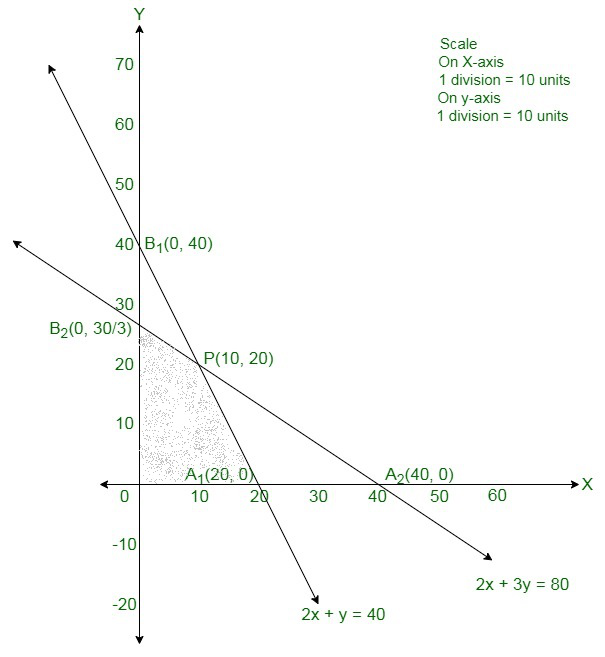The corner points are A1(20, 0), P(10, 20), B2(0, 80/3).

The value of Z = 15x + 10y at these corner points are

The maximum value of Z is 350 which is acquired at P(10, 20).

Therefore, maximum profit is attained when 10 units of deluxe model and 20 units of ordinary model is produced.

### Each machine is available for a maximum of 6 hours per day. If the profit on each cup A is 75 paise and that on each cup B is 50 paise, show that 15 tea-cups of type A and 30 of type B should be manufactured in a day to get the maximum profit.

Solution:

Assume the needed number of tea cups of Type A and B are x and y respectively.

As, the profit on each cup A is 75 paise and that on each cup B is 50 paise.

So, the profit on x tea cup of type A and y tea cup of type B are 75x and 50y respectively.

Assume total profit on tea cups be Z, so

Z = 75x + 50y

Since, every tea cup of type A and B needs to work machine I for 12 and 6 minutes respectively.

So, x tea cups of Type A and y tea cups of Type B needs to work on machine I for 12x and 6y minutes.

Total time available on machine I is 6 × 60 = 360 minutes.

As

12x + 6y ≤ 360 {First Constraint}

Thus, every tea cup of type A and B need to work machine II for 18 and 0 minutes respectively.

So, x tea cups of Type A and y tea cups of Type B need to work on machine IIII for

18x and 0y minutes respectively.

Total time available on machine I is 6 × 60 = 360 minutes.

So,

18x + 0y ≥ 360

x ≤ 20 {Second Constraint}

As, every tea cup of type A and B need to work machine III for 6 and 9 minutes respectively

So, x tea cups of Type A and y tea cups of Type B need to work on machine I

for 6x and 9y minutes respectively.

Total time available on machine I is 6 × 60 = 360 minutes.

So,

6x + 9y ≤ 360 {Third Constraint}

Therefore, the formula of the given linear programming problem is

Max Z = 75x + 50y

Subject to constraints,

12x + 6y ≤ 360

x ≤ 20

6x + 9y ≤ 360

x, y ≥ 0 {Since production of tea cups can not be less than zero}

Area 12x + 6y ≤ 360: line 12x + 6y = 360 connects axes at A(30, 0), B(0, 60)

The area having origin shows 12x + 6y ≤ 360 as (0, 0) assure 12x + 6y ≤ 360

Area x ≤ 20: line x = 20 is parallel to y-axis and connects x-axes at C(20, 0).

The area having origin shows x ≤ 20

As (0, 0) assure x ≤ 20.

Area 6x + 9y ≤ 360: line 6x + 9y = 360 connects axes at E(60, 0), F(0, 40) respectively.

The area having origin shows 6x + 9y ≤ 360 as (0, 0) assure 6x + 9y ≤ 360.

Area x, y ≥ 0: it shows the first quadrant.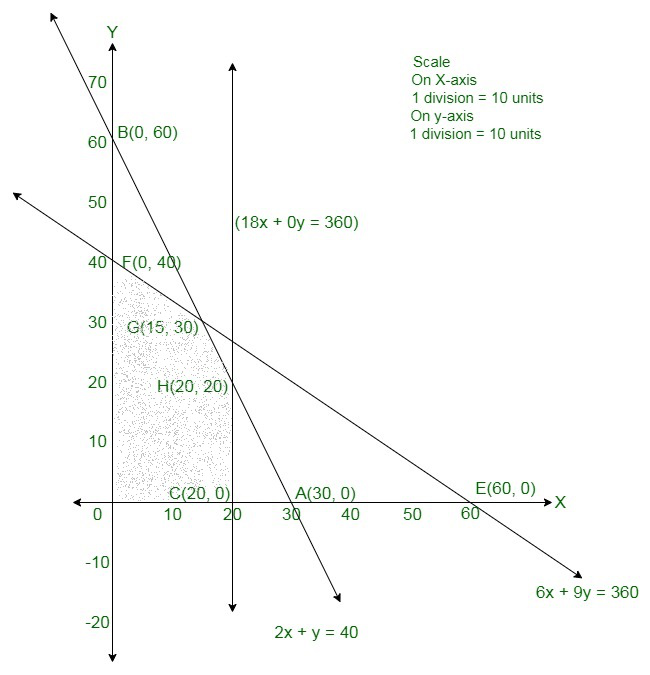The shaded area is the suitable area determined by the constraints,

12x + 6y ≤ 360

x ≤ 20

6x + 9y ≤ 360

x, y ≥ 0

The corner points are F(0, 40), G(15, 30), H(20, 20), C(20, 0).

The values of Z at these corner points are as follows

Here Z is maximum at G(15, 30).

Hence, 15 teacups of Type A and 30 tea cups of Type B are required to maximize the profit.

### He has an area of 7600 sq. m available and 72 skilled men who can operate the machines. How many machines of each type should he buy to maximize the daily output?

Solution:

Assume needed number of machine A and B are x and y respectively.

Thus, products of each machine A and B are 60 and 40 units daily respectively.

As, production by x number of machine A and y number of machine B are 60x and 40y respectively.

Assume Z is total output daily,

So,

Z = 60x + 40y

As, every machine of type A and B needs 1000sq. m and 1200 s. m area so,

x machine of type A and y machine of type B require 1000x and 1200y sq. m area but,

Total available area for machine is 7600 sq. m.

So,

1000x + 1200y ≤ 7600

or,

5x + 6y ≤ 38. {First Constraint}

As, every machine of type A and B needs 12 men and 8 men to work respectively.

So, x machine of type A and y machine of type B need 12x and 8y men to work respectively.

But total men available for work are 72.

So,

12x + 8y ≤ 72

3x + 2y ≤ 18 {Second Constraint}

Therefore, the formula of the given linear programming problem is

Max Z = 50x + 40y

Subject to constraints,

5x + 6y ≤ 38

3x + 2y ≤ 18

x, y ≥ 0 {Since number of machines can not be less than zero}

Area 5x + 6y ≤ 38: line 5x + 6y = 38 connects the axes at A(38/5, 0), B(0, 19/3) respectively.

Area having the origin shows 5x + 6y ≤ 38 as origin satisfies 5x + 6y ≤ 38

Area 3x + 2y ≤ 18: line 3x + 2y = 18 connects the axes at C(6, 0), D(0, 9) respectively.

Area having the origin shows 3x + 2y ≤ 18 as origin assure 3x + 2y ≤ 18.

Area x, y ≥ 0: it represents the first quadrant.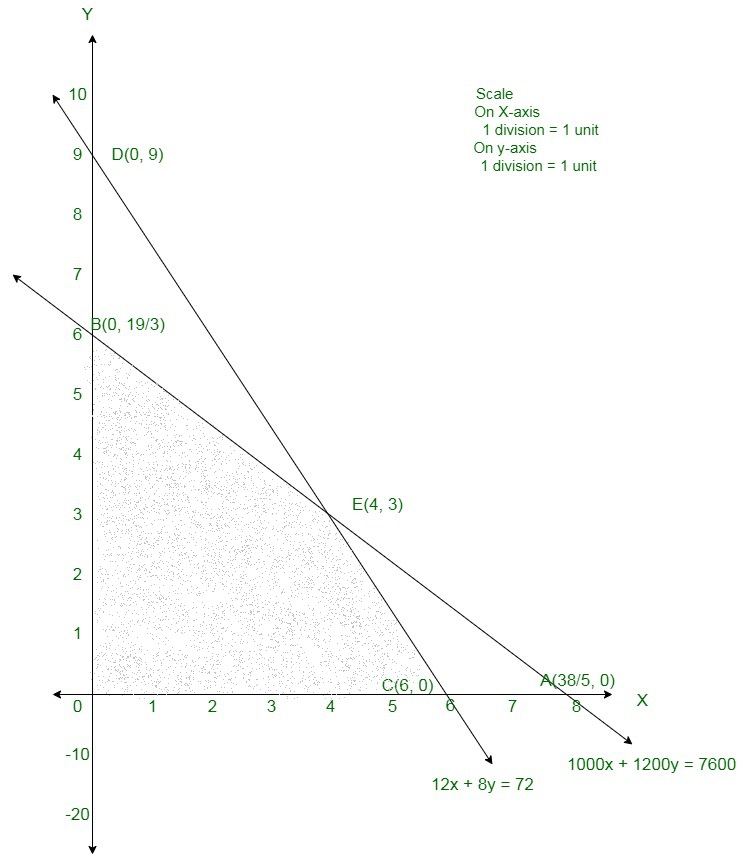Shaded region shows the suitable area.

The corner points are O(0, 0), B(0, 19/3), E(4, 3), C(6, 0).

Therefore, the values of Z at these corner points are as follows:

The maximum value of Z is 360 which is acquired at E(4, 3), C(6, 0).

Therefore, the maximum output is Rs 360 archive when 4 units of type A and

3 units of type B or 6 units of type A and 0 units of type B are manufactured.

### Question 9. A company produces two types of goods, A and B, that require gold and silver. Each unit of type A requires 3 gm of silver and 1 gm of gold while that of type B requires 1 gm of silver and 2 gm of gold. The company can produce 9 gm of silver and 8 gm of gold. If each unit of type A brings a profit of Rs 40 and that of type B Rs 50, find the number of units of each type that the company should produce to maximize the profit. What is the maximum profit?

Solution:

Assume needed number of goods A and B are x and y respectively.

As, profits of each A and B are Rs. 40 and Rs. 50 respectively.

Thus, profits on x number of type A and y number of type B are 40x and 50y respectively.

Assume Z is total output daily,

So,

Z = 40x + 50y

So, every A and B needs 3 grams and 1 gram of silver respectively.

Thus, x of type A and y of type B need 3x and y of silver respectively.

But,

Total silver available is 9 grams. So,

3x + y

9 {First Constraint}

So, every A and B needs 1 gram and 2 grams of gold respectively.

Thus, x of type A and y of type B require x and 2y respectively.

But total gold available is 8 grams.

Thus,

x + 2y ≤ 8 {Second Constraint}

Therefore, the formula of the given linear programming problem is

Max Z = 40x + 50y

Subject to constraints,

3x + y ≤ 9

x + 2y ≤ 8

x, y ≥ 0 {Since production of A and B can not be less than zero}

Area 3x + y ≤ 9: line 3x + y = 9 connects the axes at A(3, 0), B(0, 9) respectively.

The area having the origin shows 3x + y ≤ 9

As, origin assure 3x + y ≤ 9.

Area x + 2y ≤ 8: line x + 2y = 8 connects the axes at C(8, 0), D(0, 4) respectively.

Area having the origin shows x + 2y ≤ 8 as origin assure x + 2y ≤ 8.

Area x, y ≥ 0: it shows the first quadrant.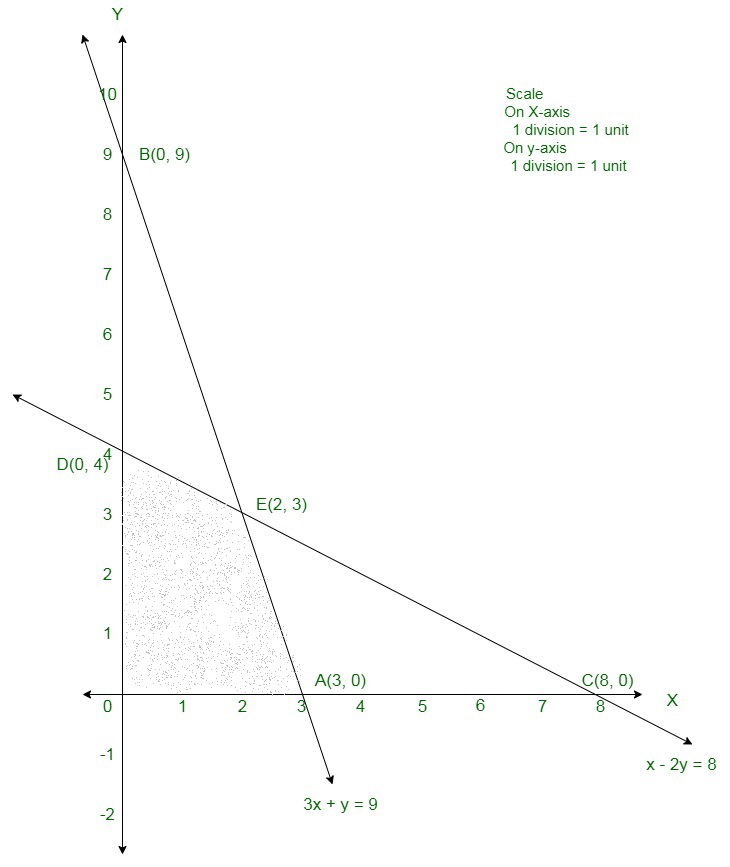The corner points are O(0, 0), D(0, 4), E(2, 3), A(3, 0)

The values of Z at these corner points are as follows

The maximum value of Z is 230 which is obtained at E(2, 3).

Therefore, the maximum profit is of Rs 230 when 2 units of Type A 3 units of Type B are produced.

### Question 10. A manufacturer of Furniture makes two products: chairs and tables. Processing of these products is done on two machines A and B. A chair requires 2 hrs on machine A and 6 hrs on machine B. A table requires 4 hrs on machine A and 2 hrs on machine B. There are 16 hrs 0of time per day available on machine A and 30 hrs on machine B. Profit gained by the manufacturer from a chair and a table is Rs 3 and Rs 5 respectively. Find with the help of graph what should be the daily production of each of the two products so as to maximize his profit.

Solution:

Assume daily production of chairs and tables be x and y respectively.

So, profits of every chair and table is Rs. 3 and Rs. 5 respectively.

Thus, profits on x number of type A and y number of type B are 3x and 5y respectively.

Assume Z is total output daily,

So,

Z = 3x + 5y

As, every chair and table needs 2 hrs and 3 hrs on machine A respectively.

So, x number of chair and y number of table need 2x and 4y hrs on machine A respectively.

But,

Total time available on Machine A is 16 hours.

So,

2x + 3y ≤ 16

x + 2y ≤ 8 {First Constraint}

So, every chair and table needs 6 hrs and 2 hrs on machine B respectively.

Since, x number of chair and y number of table need 6x and 2y hrs on machine B respectively.

But,

Total time need on Machine B is 30 hours. So,

6x + 2y ≤ 30

3x + y ≤ 15 {Second Constraint}

Therefore, the formula of the given linear programming problem is

Max Z = 3x + 5y

Subject to constraints,

x + 2y ≤ 8

3x + y ≤ 15

x, y ≥ 0 {Since production of chairs and tables can not be less than zero}

Area x + 2y ≤ 8: line x + 2y = 8 connects the axes at A(8, 0), B(0, 4) respectively.

Area having the origin shows x + 2y ≤ 8

As, origin assure x + 2y ≤ 8.

Area 3x + y ≤ 15: line 3x + y = 15 connects the axes at C(5, 0), D(0, 15) respectively.

Area having the origin shows 3x + y ≤ 15 as origin assure 3x + y ≤ 15

Area x, y ≥ 0: it shows the first quadrant.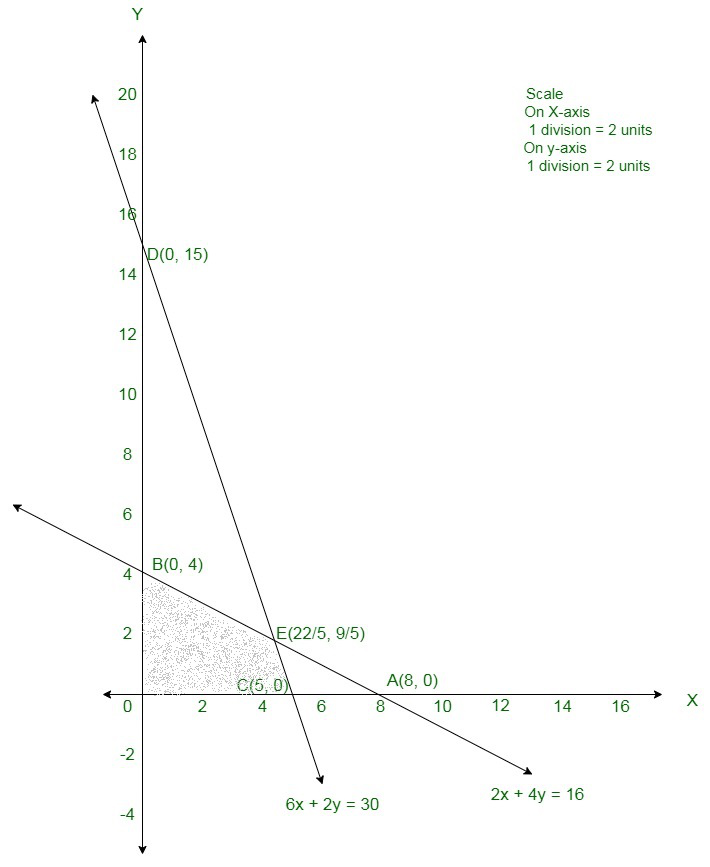The corner points are O(0, 0), B(0, 4), E(22/5, 9/5), and C(5, 0).

The values of Z at these corner points are as follows,

The maximum value of Z is 22.2 which is obtained at E(22/5, 9/5).

Therefore, the maximum profit of Rs 22.2 when 22/5 units of chair and 9/5 units of table are produced.

### Question 11. A furniture manufacturing company plans to make two products: chairs and tables. From its available resources which consists of 400 square feet to teak wood and 450 man-hours. It is known that to make a chair requires 5 square feet of wood and 10 man-hours and yield sa profit of Rs 45, while each table uses 20 square feet of wood and 25 man-hours and yields a profit of Rs 80. How many items of each product should be produced by the company so that the profit is maximum?

Solution:

Assume needed production of chairs and tables be x and y respectively.

As, profits of every chair and table is Rs. 45 and Rs. 80 respectively.

Thus, profits on x number of type A and y number of type B are 45x and 80y respectively.

Assume Z is total output daily,

So, Z = 45x + 80y

As, every chair and table needs 5 sq. ft and 80 sq. ft of wood respectively.

As, x number of chair and y number of table need 5x and 80y sq. ft of wood respectively.

But, 400 sq. ft of wood is accessible.

So, 5x + 80y ≤ 400

x + 4y ≤ 80 {First Constraint}

As, every chair and table needs 10 and 25 men – hours respectively.

As, x number of chair and y number of table need 10x and 25y men – hours respectively.

But,

Only 450 hours are available .

So,

10x + 25y ≤ 450

2x + 5y ≤ 90 {Second Constraint}

Therefore, the formula of the given linear programming problem is

Max Z = 45x + 80y

Subject to constraints,

x + 4y ≤ 80

2x + 5y ≤ 90

x, y ≥ 0 {Since production of chairs and tables can not be less than zero}

Area x + 4y ≤ 80: line x + 4y = 80 connects the axes at A(80, 0), B(0, 20) respectively.

Area having the origin shows x + 4y ≤ 80 as origin assure x + 4y ≤ 80

Area 2x + 5y ≤ 90: line 2x + 5y = 90 connects the axes at C(45, 0), D(0, 20) respectively.

Area having the origin shows 2x + 5y ≤ 90

As origin assure 2x + 5y ≤ 90

Area x, y ≥ 0: it shows the first quadrant.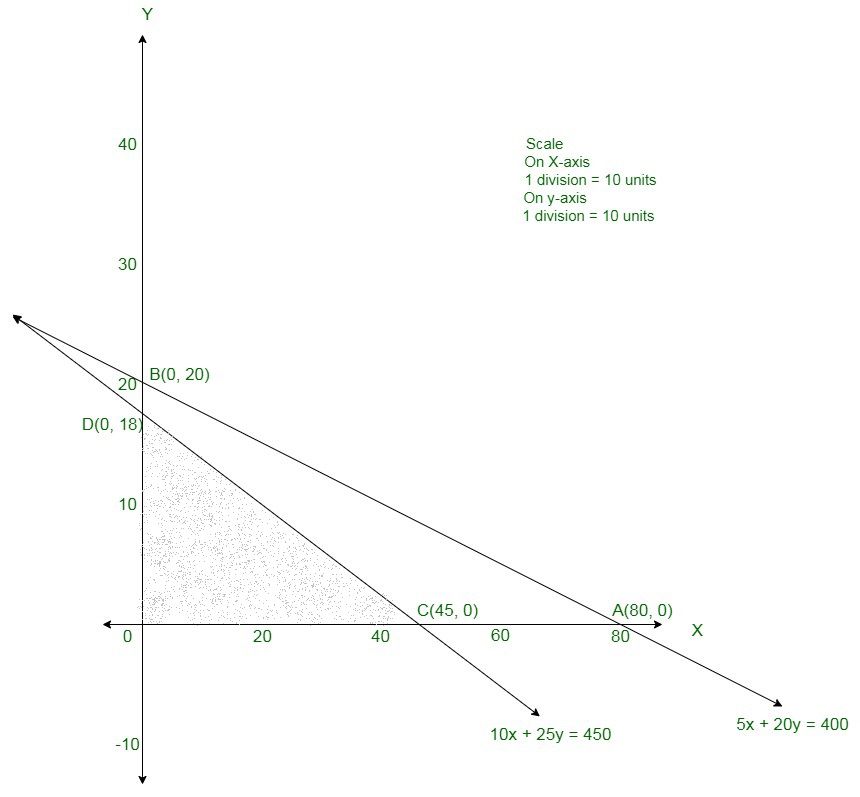The corner points are O(0, 0), D(0, 18), C(45, 0).

The values of Z at these corner points are as follows:

The maximum value of Z is 2025 which is obtained at C(45, 0).

Therefore, the maximum profit of Rs 2025 is attained when 45 units of chairs and no units of tables are produced.

### Question 12. A firm manufactures two products A and B. Each product is processed on two machines M1 and M2. Product A requires 4 minutes of processing time on M1 and 8 min. on M2 ; product B requires 4 minutes on M1 and 4 min. on M2. The machine M1 is available for not more than 8 hrs 20 min. while machine M2 is available for 10 hrs. during any working day. The products A and B are sold at a profit of Rs 3 and Rs 4 respectively. Formulate the problem as a linear programming problem and find how many products of each type should be produced by the firm each day in order to get maximum profit and solve it graphically.

Solution:

Assume needed production of product A and B be x and y respectively.

As, profit on every product A and B are Rs. 3 and Rs. 4 respectively.

Thus, profits on x number of type A and y number of type B are 3x and 4y respectively.

Assume Z is total output daily,

So,

Z = 3x + 4y

Thus, every A and B needs 4 minutes each on machine M1

As, x of type A and y of type B need 4x and 4y minutes respectively.

But,

Total time accessible on machine M1 is 8 hours 20 minutes = 500 minutes.

Thus,

4x + 4y ≤ 500

x + y ≤ 125 {First Constraint}

So, every A and B needs 8 minutes and 4 minutes on machine M2 respectively.

Thus, x of type A and y of type B need 8x and 4y minutes respectively.

But,

Total time accessible on machine M1 is 10 hours = 600 minutes.

Thus,

8x + 4y ≤ 600

2x + y ≤ 150 {Second Constraint}

Therefore, the formula of the given linear programming problem is

Max Z = 3x + 4y

Subject to constraints,

x + y ≤ 125

2x + y ≤ 150

x, y ≥ 0 {Since production of A and B can not be less than zero}

Area x + y ≤ 125: line x + y = 125 connects the axes at A(125, 0), B(0, 125) respectively.

Area having the origin shows x + y ≤ 125 as origin assure x + y ≤ 125.

Area 2x + y ≤ 150: line 2x + y = 150 connects the axes at C(75, 0), D(0, 150) respectively.

Area having the origin shows 2x + y ≤ 150 as origin assure 2x + y ≤ 150.

Area x, y ≥ 0: it shows the first quadrant.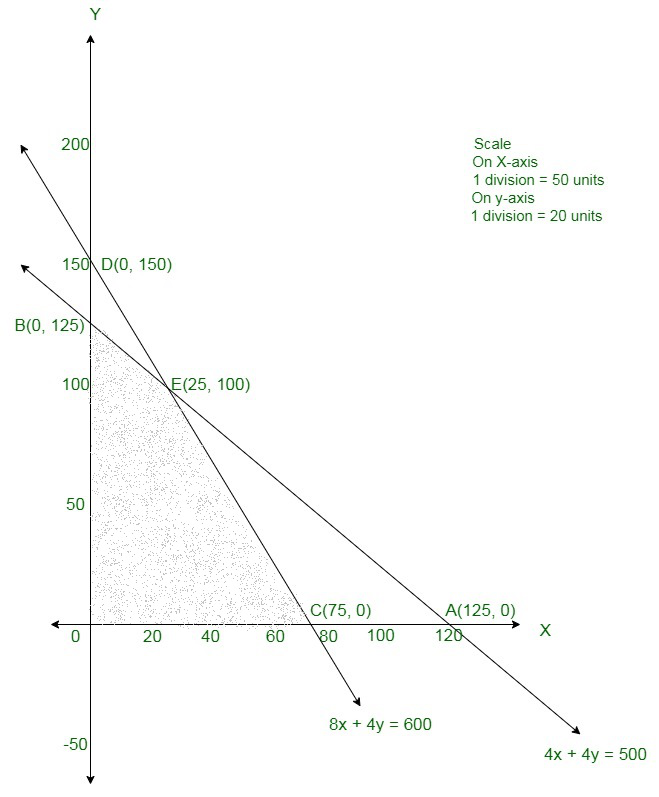The corner points are O(0, 0), B(0, 125), E(25, 100), and C(75, 0).

The values of Z at these corner points are as follows:

The maximum value of Z is 500 which is obtained at B(0, 125).

Therefore, the maximum profit is Rs 500 attained when no units of product A and

125 units of product B are manufactured.

### Question 13. A firm manufacturing two types of electric items, A and B, can make a profit of Rs 20 per unit of A and Rs 30 per unit of B. Each unit of A requires 3 motors and 4 transformers and each unit of B requires 2 motors and 4 transformers. The total supply of these per month is restricted to 210 motors and 300 transformers. Type B is an export model requiring a voltage stabilizer which has a supply restricted to 65 units per month. Formulate the linear programming problem for maximum profit and solve it graphically.

Solution:

Assume x units of item A and y units of item B were manufactured.

Numbers of items cannot be negative.

Therefore,

x, y ≥ 0 (always)

Given: The information can be tabulated as follows:

Thus, after this it is given that type B is an export model, whose supply is restricted to 65 units per month.

So,

The constraints are

3x + 2y ≤ 210

4x + 4y ≤ 300

y ≤ 65

A and B can make profit of Rs 20 and Rs 30 per unit respectively.

Hence, profit gained from x units of item A and y units of item B is Rs 20x and 30y respectively.

Assume total profit be Z,

So,

Total Profit = Z = 20x + 30y as per the question is to be maximised.

Therefore, the formula of the given linear programming problem is

Max Z = 20x + 30y

Subject to constraints

3x + 2y ≤ 210

4x + 4y ≤ 300

y ≤ 65

x, y ≥ 0

Area shown by 3x + 2y ≤ 210: The line 3x + 2y = 210 connects the axes at A(70, 0), B(0, 105) respectively.

Area having the origin shows 3x + 2y ≤ 210 as origin assure 3x + 2y ≤ 210.

Area shown by 4x + 4y ≤ 300: The line 4x + 4y = 300 connects the axes at C(75, 0), D(0, 75) respectively.

Area having the origin shows 4x + 4y ≤ 300 as origin assure 4x + 4y ≤ 300

y = 65 is the line passing through the point E(0, 65) and is parallel to x – axis.

Area x,y ≥ 0: it shows the first quadrant.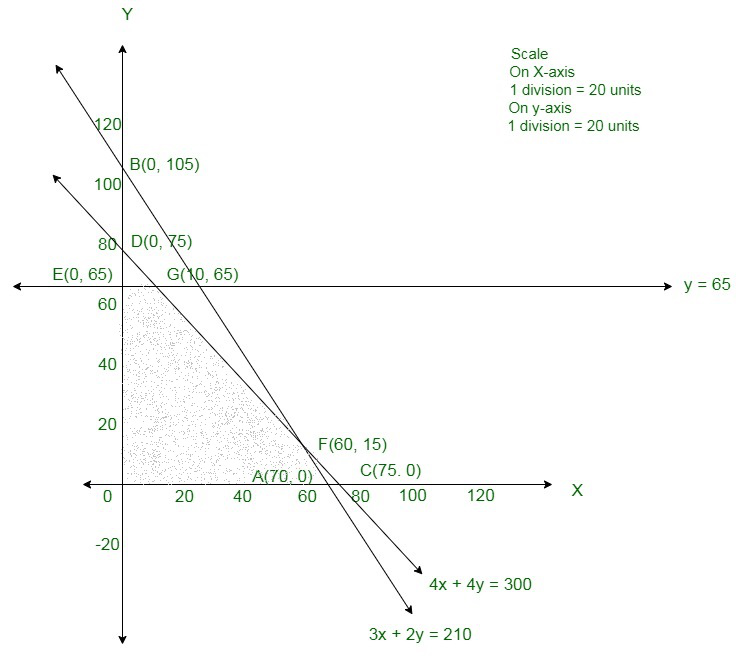The corner points are O(0, 0), E(0, 65), G(10, 65), F(60, 15) and A(70, 0).

The values of Z at these corner points are as follows:

The maximum value of Z is 2150 which is obtained at G(10, 65).

Therefore, the maximum profit is Rs. 2150 attained when 10 units of item A and

65 units of item B are manufactured.

### Question 14. A factory uses three different resources for the manufacture of two different products, 20 units of resources A, 12 units of B and 16 units of C being available. 1 unit of the first product requires 2, 2 and 4 units of the respective resources and 1 unit of the second product requires 4, 2, and 0 units of respective resources. It is known that the first product gives a profit of 2 monetary units per unit and the second 3. Formulate the linear programming problem. How many units of each product should be manufactured to maximize the profit? Solve it graphically.

Solution:

Assume number of product I and product II are x and y respectively.

As, profits on every product I and II are 2 and 3 monetary unit.

As, profits on x number of Product I and y number of Product II are 2x and 3y respectively.

Assume Z is total output daily,

So,

Z = 2x + 3y

Here, every I and II needs 2 and 4 units of resources A.

So, x units of product I and y units of product II needs 2x and 4y minutes respectively.

But, maximum available quantity of resources A is 20 units.

So,

2x + 4y ≤ 20

x + 2y ≤ 10 {First Constraint}

So, each I and II needs 2 and 2 units of resources B.

So, x units of product I and y units of product II needs 2x and 2y minutes respectively.

But, maximum available quantity of resources A is 12 units.

So,

2x + 2y ≤ 12

x + y ≤ 6 {Second Constraint}

Here, every unit of product I require 4 units of resources C.

It is not needed by product II. Thus, x units of product I require 4x units of resource C.

But, maximum available quantity of resources C is 16 units.

Thus,

4x ≤ 16

x ≤ 4 {Third Constraint}

Therefore, the formula of the given linear programming problem is

Max Z = 2x + 3y

Subject to constraints,

x + 2y ≤ 10

x + y ≤ 6

x ≤ 4

x, y ≥ 0  {Since production for I and II can not be less than zero}

Area shown by x + 2y ≤ 10: The line x + 2y = 10 connects the axes at A(10, 0), B(0, 5) respectively.

Area having the origin shows x + 2y ≤ 10 as origin assure x + 2y ≤ 10.

Area shown by x + y ≤ 6: The line x + y = 6 connects the axes at C(6, 0), D(0, 6) respectively.

Area having the origin shows x + y ≤ 6 as origin assure x + y ≤ 6

Area x, y ≥ 0: it shows the first quadrant.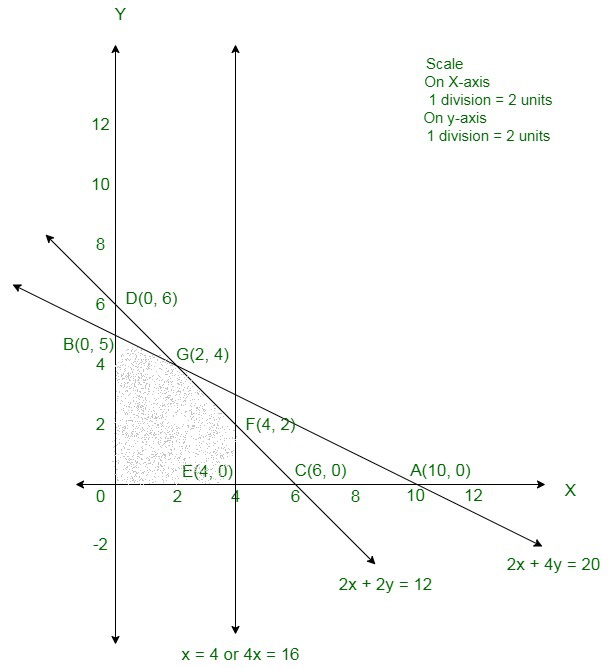The corner points are O(0, 0), B(0, 5), G(2, 4), F(4, 2), and E(4, 0).

The values of Z at these corner points are as follows:

The maximum value of Z is 16 which is obtained at G (12, 4).

Therefore, the maximum profit is 16 monetary units attained when 2 units of first product and

4 units of second product were manufactured.

### Question 15. A publisher sells a hard cover edition of a text book for Rs 72.00 and paperback edition of the same ext for Rs 40.00. Costs to the publisher are Rs 56.00 and Rs 28.00 per book respectively in addition to weekly costs of Rs 9600.00. Both types require 5 minutes of printing time, although hardcover requires 10 minutes binding time and the paperback requires only 2 minutes. Both the printing and binding operations have 4,800 minutes available each week. How many of each type of book should be produced in order to maximize profit?

Solution:

Assume the sale of hand cover edition be ‘h’ and that of paperback editions be ‘t’.

SP of a hard cover edition of the textbook = Rs 72

SP of a paperback edition of the textbook = Rs 40

Cost to the publisher for hard cover edition = Rs 56

Cost to the publisher for a paperback edition = Rs 28

Weekly cost to the publisher = Rs 9600

Profit to be maximized, Z = (72 – 56)h + (40 – 28)t – 9600

Z = 16h + 12t – 9600

5(h + t) ≤ 4800

10h + 2t ≤ 4800.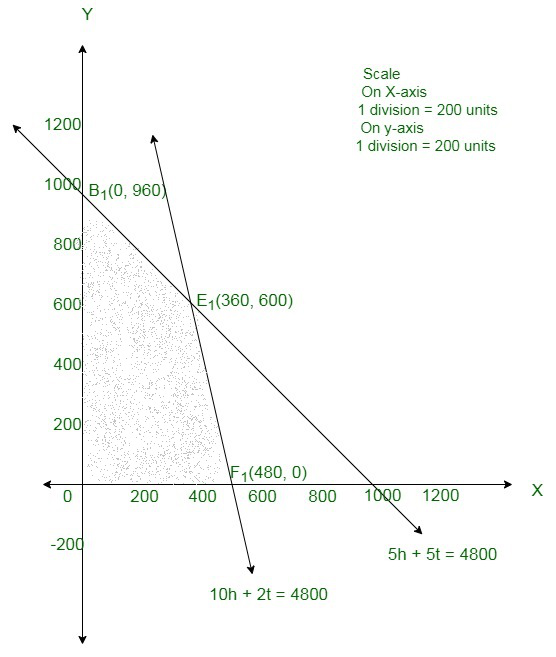The corner points are O(0, 0), B1(0, 960), E1 (360, 600) and F1 (480, 00).

The values of Z at these corner points are as follows:

The maximum value of Z is 3360 which is obtained at E1 (360, 600).

Hence, the maximum profit is 3360 which is attained by selling 360 copies of hardcover edition and

600 copies paperback edition.

### Question 16. A firm manufactures headache pills in two sizes A and B. Size A contains 2 grains of aspirin, 5 grains of bicarbonate and 1 grain of codeine; size B contains 1 grain of aspirin, 8 grains of bicarbonate and 66 grains of codeine. It has been found by users that it requires at least 12 grains of aspirin, 7.4 grains of bicarbonate and 24 grains of codeine for providing immediate effects. Determine graphically the least number of pills a patient should have to get immediate relief. Determine also the quantity of codeine consumed by patient.

Solution:

Here the above LPP can be shown as a table below,

Therefore, the formula of the given linear programming problem is

Max Z = x + y

Subject to constraints,

2x + y  ≥ 12

5x + 8y ≥ 7.4

x + 66y ≥ 24

x, y ≥ 0  {Since production can not be less than zero}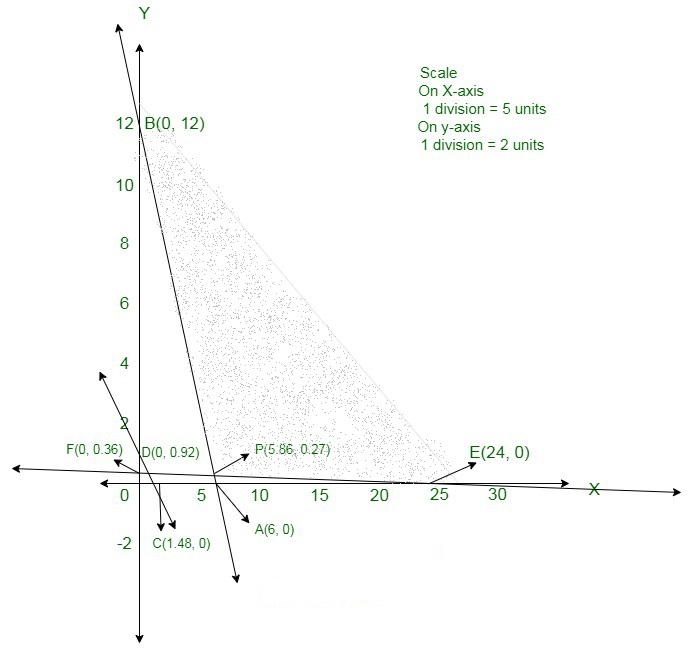The corner points are B(0, 12), P(5.86, 0.27), E(24, 0)

The values of Z at these corner points are as follows:

The minimum value of Z is 6.13.

But,

The area is unbounded thus check whether x + y < 6.13

Thus, we can see that it does not has any common area.

So, x = 5.86, y = 0.27

This is the least quantity of pill A and B.

Codeine quantity = x + 66y = 5.86 + (66 × 0.27) = 24(approx).

### Question 17. A chemical company produces two compounds, A and B. The following table gives the units of ingredients, C and D per kg of compounds A and B as well as minimum requirements of C and D and costs per kg of A and B. Find the quantities of A and B which would give a supply of C and D at a minimum cost.

Solution:

Assume needed quantity of compound A and B are x and y kg.

As, cost of one kg of compound A and B are Rs 4 and Rs 6 per kg. So,

Cost of x kg of compound A and y kg of compound B are Rs 4x and Rs 6y

Respectively.

Assume Z be the total cost of compounds,

So,

Z = 4x + 6y

As, compound A and B contain 1 and 2 units of ingredient C per kg respectively,

Thus, x kg of compound A and y kg of compound B contain x and 2y units of

ingredient C respectively but minimum requirement of ingredient C is 80 units,

So,

x + 2y ≥ 80 {first constraint}

Hence, compound A and B have 3 and 1 units of ingredient D per kg respectively,

Hence, x kg of compound A and y kg of compound B have 3x and y units of

ingredient D respectively but minimum need of ingredient C is 75 units, so,

3x + y ≥ 75 {second constraint}

Therefore, the formula of the given linear programming problem is

Min Z = 4x + 6y

Subject to constraints,

x + 2y ≥ 80

3x + y ≥ 75

x, y ≥ 0 {Since production can not be less than zero}

Area x + 2y ≥ 80: line x + 2y = 80 connects axes at A(80, 0), B(0, 40) respectively.

Area not having origin shows x + 2y ≥ 80 as (0, 0) does not assure x + 2y ≥ 80.

Area 3x + y ≥ 75: line 3x + y = 75 connects axes at C(25, 0), D(0, 75) respectively.

Area not having origin shows 3x + y ≥ 75 as (0, 0) does not assure 3x + y ≥ 75.

Area x,y ≥ 0: it shows first quadrant.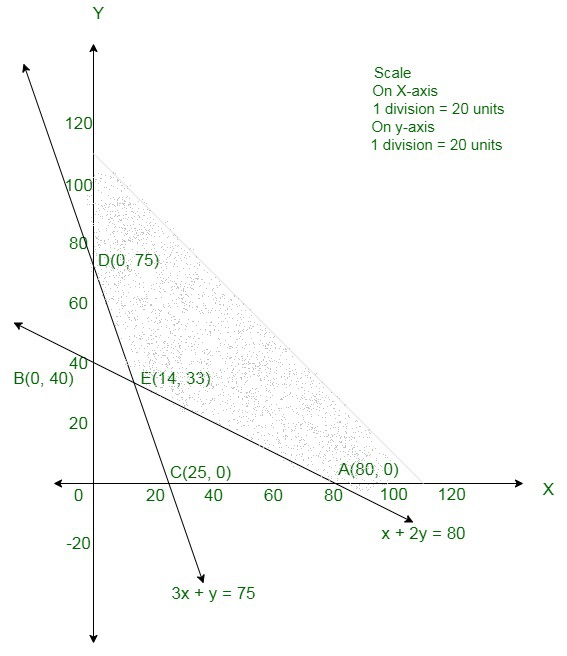The corner points are D(0, 75), E(14, 33), A(80, 0).

The values at Z at these corner points are as follows:

The minimum value of Z is 254 which is obtained at E(14, 33).

Therefore, the minimum cost is Rs 254 attained when 14 units of compound A and

33 units compound B are produced.

My Personal Notes arrow_drop_up